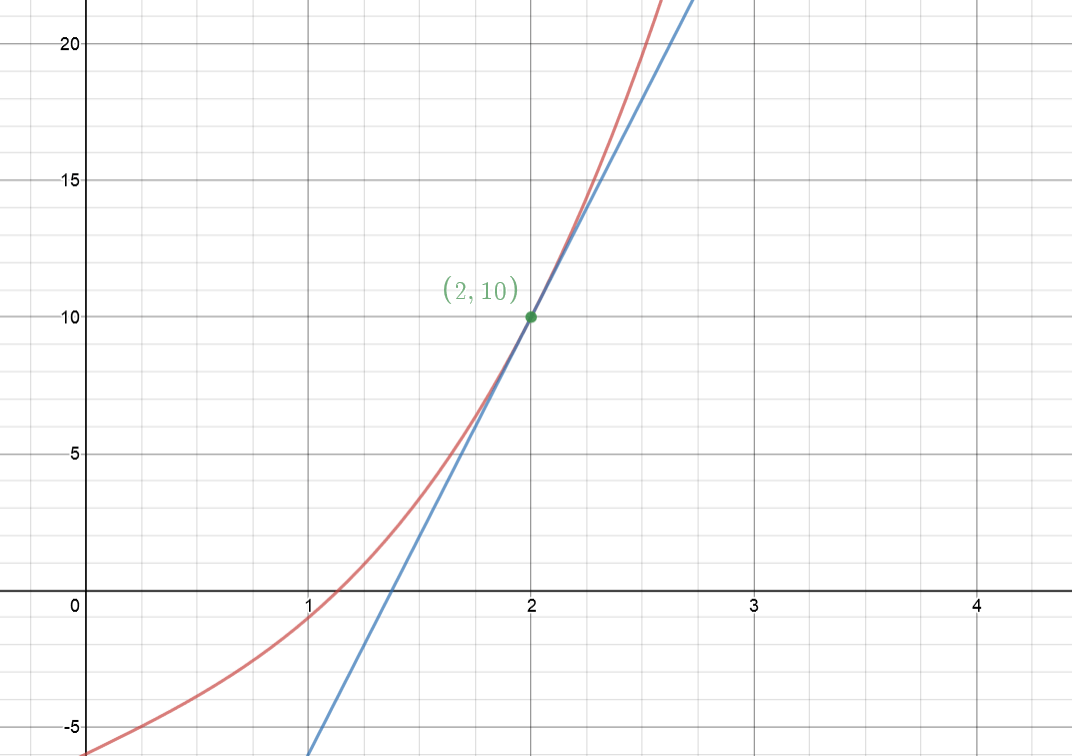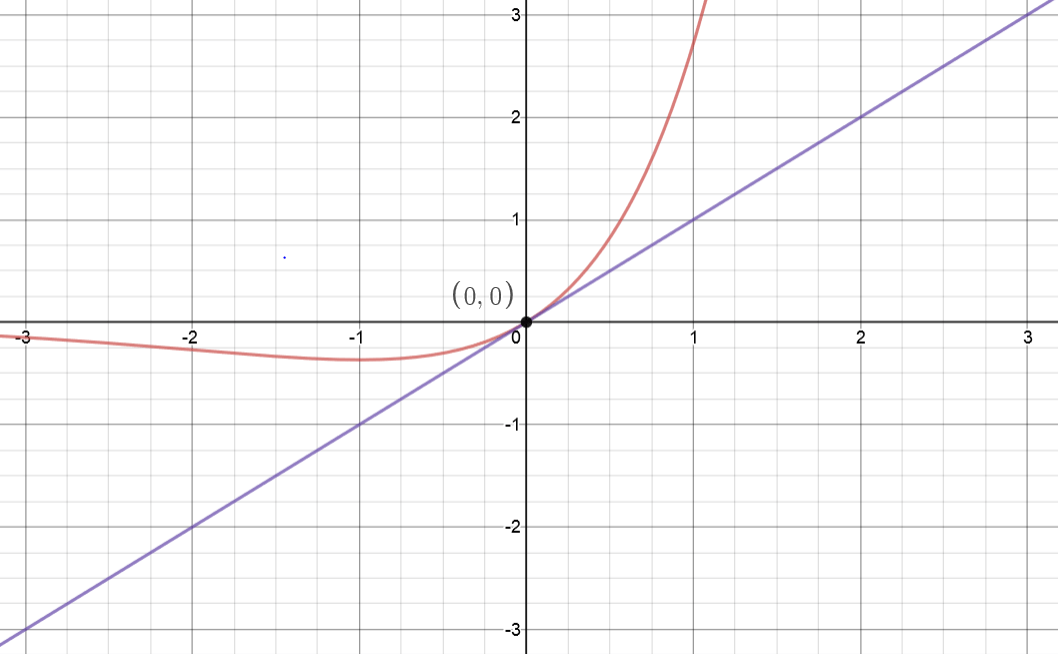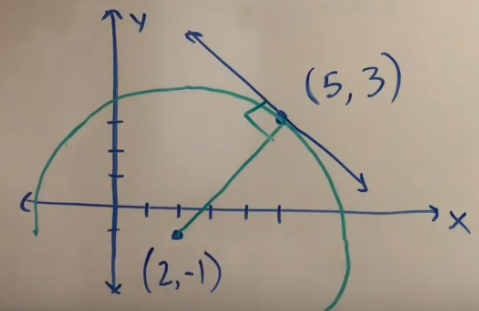## How to find the equation of a tangent line

One common application of the derivative is to find the equation of a tangent line to a function. Usually when you’re doing a problem like this, you will be given a function whose tangent line you need to find. And you will also be given a point or an x value where the line needs to be tangent to the given function.

Using these two pieces of information, you need to create an equation for a line that satisfies the required conditions. This process is very closely related to linear approximation (or linearization) and differentials.

When coming up with the equation of the line, there are a couple different approached you could take. You should decide which one to use based on your own personal preference. The only difference between the different approaches is which template for an equation of a line you prefer to use. Remember, there are two main forms that a line will take: $$y=mx+b$$ $$y=m(x-x_0)+y_0$$ Another thing to keep in mind is that the first form is generally easier when we are given the y-intercept of the line. The second form above is usually easier when we are given any other point that isn’t the y-intercept.

In both of these forms, x and y are variables and m is the slope of the line. In the first equation, b is the y-intercept. And in the second equation, $$x_0$$ and $$y_0$$ are the x and y coordinates of some point that lies on the line. This could be any point that lies on the line.

It is also important to notice that a line would be tangent to a function at a specific point if and only if the following two conditions are met.

• The function and its tangent line need to go through the same point
• and they both need to share the same slope at that shared point.

## But how does the derivative apply?

You should always keep in mind that a derivative tells you about the slope of a function. So if we take a function’s derivative, then look at it at a certain point, we have some information about the slope of the function at that point. Since a tangent line has to have the same slope as the function it’s tangent to at the specific point, we will use the derivative to find m.

So let’s jump into a couple examples and I’ll show you how to do something like this.

## Example 1

Find the equation of the tangent line to the function $$\mathbf{y=x^3+4x-6}$$ at the point (2, 10).

In order to find this tangent line, let’s consider the two conditions that need to be met for our line to be a tangent line at the specified point.

1. The tangent line and the given function need to go through the same point. Since the problem told us to find the tangent line at the point $$(2, \ 10)$$, we know this will be the point that our line has to go through.
2. The tangent line and the function need to have the same slope at the point $$(2, \ 10)$$. In order to find this slope we will need to use the derivative. Let’s start with this.

#### Finding the slope of the tangent line

Remember that the derivative of a function tells you about its slope. So to find the slope of the given function $$y=x^3+4x-6$$ we will need to take its derivative. This will just require the use of the power rule. $$y’=3x^2+4$$

But how can we use this to find the slope of the tangent line when it has variables in it?

This is where the specific point we need to consider comes into play. We know that the tangent line and the function need to have the same slope at the point $$(2, \ 10)$$. Therefore, they need to have the same slope when $$x=2$$. In order to find the slope of the given function y at $$x=2$$, all we need to do is plug 2 into the derivative of y.

Therefore, the slope of our line would simply be $$y'(2)=3(2)^2+4=16.$$ And because of this we also know the slope of our tangent line will be $$m=16.$$ So we know this will guarantee that our tangent line has the right slope, now we just need to make sure it goes through the right point.

#### Making sure the tangent line contains the given point

Since we do know a point that has to lie on our line, but don’t know the y-intercept of the line, it would be easier to use the following form for our tangent line equation. $$y=m(x-x_0)+y_0$$

And since we already know $$m=16$$, let’s go ahead and plug that into our equation. $$y=16(x-x_0)+y_0$$

Now to finish our tangent line equation, we just need the x and y coordinates of a point that lies on this line. Then we can simply plug them in for $$x_0$$ and $$y_0$$. Well, we were given this information! We were told that the line we come up with needs to be tangent at the point $$(2, \ 10)$$. Therefore, our tangent line needs to go through that point. This tells us our tangent line equation must be $$y=16(x-2)+10$$ $$y=16x-32+10$$ $$y=16x-22$$

And that’s it! We know that the line $$y=16x-22$$ will go through the point $$(2, 10)$$ on our original function. And we know that it will also have the same slope as the function at that point.

We can even use Desmos to check this and see what our function and tangent line look like together.$$y=x^3+4x-6$$ and $$y=16x-22$$

## Example 2

Find the equation of the line that is tangent to the function $$f(x) = xe^x$$ when $$x=0$$.

To start a problem like this I suggest thinking about the two conditions we need to meet.

1. The tangent line and the given function need to intersect at $$\mathbf{x=0}$$. This time we weren’t given the y coordinate of this point so we will need to figure that out.
2. Then we need to make sure that our tangent line has the same slope as f(x) when $$\mathbf{x=0}$$.

#### Finding the slope of the tangent line

I personally think that it’s a little easier to find the slope of the tangent line first, but you can start with making sure the other condition is met if you prefer.

When you’re asked to find something to do with slope, your first thought should be to use the derivative. The derivative of a function tells you about it’s slope. Since we need the slope of f(x), we’ll need its derivative.

Looking at our function $$f(x)=xe^x$$ you can see that it is the product of two simpler functions. To find it’s derivative we will need to use the product rule. I’m not going to show every step of this, but if you aren’t 100% sure how to find this derivative you should click the link in the last sentence. $$f'(x) = e^x + xe^x$$ $$f'(x) = e^x \big(1+x \big)$$

Now consider the fact that we need our tangent line to have the same slope as f(x) when $$x=0$$. To find the slope of f(x) at $$x=0$$ we just need to plug in 0 for x into the equation we found for f'(x). $$f'(0) = e^{(0)} \big( 1 + (0) \big)$$ $$f'(0) = 1(1)=1$$

So we know that the slope of our tangent line needs to be 1.

#### Making sure the tangent line contains the required point

Now we just need to make sure that our tangent line shares the same point as the function when $$x=0$$. In order to do this, we need to find the y value of the function when $$x=0$$. This would be the same as finding f(0). $$f(0) = (0)e^{(0)} = 0$$

Since this is the y value when $$x=0$$, we can also say that this is the y-intercept. We know the y intercept of our tangent line is 0. Since we figured out the y-intercept, it would be easiest to use the $$y=mx+b$$ form of the line for the tangent line equation.

We already found that the slope will be 1 and that the y-intercept will need to be 0, so we can plug these values in for m and b. Doing this tells us that the equation of our tangent line is $$y=(1)x+(0)$$ $$y=x.$$

Again, we can see what this looks like and check our work by graphing these two functions with Desmos.$$y=xe^x$$ and $$y=x$$

## Finding the Tangent Line Equation with Implicit Differentiation

Depending on the curve whose tangent line equation you are looking for, you may need to apply implicit differentiation to find the slope.

## Example 3

Find the equation of the line that is tangent to the curve $$\mathbf{y^3+xy-x^2=9}$$ at the point (1, 2).

First we need to apply implicit differentiation to find the slope of our tangent line.

$$\frac{d}{dx} \big[ y^3 + xy – x^2 \big] = \frac{d}{dx} $$ $$3y^2 \frac{dy}{dx} + 1\cdot y + x \cdot \frac{dy}{dx} – 2x = 0$$ $$3y^2 \frac{dy}{dx} + x \frac{dy}{dx} = -y + 2x$$ $$\frac{dy}{dx} \big[ 3y^2 + x \big] = -y + 2x$$ $$\frac{dy}{dx} = \frac{-y+2x}{3y^2+x}$$

Now we can plug in the given point (1, 2) into our equation for $$\mathbf{\frac{dy}{dx}}$$ to find the slope of the tangent line.

$$m=\frac{-(2)+2(1)}{3(2)^2+(1)}=\frac{0}{13}=0$$

With this slope, we can go back to the point slope form of a line. Since we know the slope and a point that lies on this line, we can plug that information into the general point slope form for a line. This will leave us with the equation for a tangent line at the given point.

$$y=m(x-x_0)+y_0$$ $$y=0(x-1)+2$$ $$y=2$$

So the constant function $$\mathbf{y=2}$$ is tangent to the curve $$\mathbf{y^3+xy-x^2=9}$$ at the point (1, 2).

## Example 4

Find the equation of the line that is tangent to the curve $$\mathbf{16x^2 + y^2 = xy + 4}$$ at the point (0, 2).

Again, we will start by applying implicit differentiation to find the slope of the tangent line.

$$\frac{d}{dx} \big[ 16x^2 + y^2 \big] = \frac{d}{dx} [xy + 4]$$ $$32x + 2y \frac{dy}{dx} = 1\cdot y + x \cdot \frac{dy}{dx}$$ $$2y \frac{dy}{dx} – x \frac{dy}{dx}= -32x + y$$ $$\frac{dy}{dx} \big[ 2y-x \big] = -32x+y$$ $$\frac{dy}{dx} = \frac{-32x+y}{2y-x}$$

Now we can plug in the given point (0, 2) into our equation for $$\mathbf{\frac{dy}{dx}}$$ to find the slope of the tangent line.

$$m = \frac{-32(0)+(2)}{2(2)-(0)}$$ $$m=\frac{2}{4}$$ $$m=\frac{1}{2}$$

With this slope, we can go back to the point slope form of a line. Since we know the slope and a point that lies on this line, we can plug that information into the general point slope form for a line. This will leave us with the equation for a tangent line at the given point.

$$y=m(x-x_0)+y_0$$ $$y=\frac{1}{2}(x-0)+2$$ $$y=\frac{1}{2}x+2$$

## Tangent Line Equation Without Derivatives

There are some cases where you can find the slope of a tangent line without having to take a derivative. This is not super common because it does require being able to take advantage of additional information. Usually you will be able to do this if you know some geometrical fact about the curve whose tangent line equation you are looking for.

The most common example of this is finding the a line that is tangent to a circle.

## Example 5

Find the equation of the line that is tangent to the circle $$\mathbf{(x-2)^2+(y+1)^2=25}$$ at the point (5, 3).

We already are given a point that we know needs to lie on our tangent line. This tells us that if we can find the slope of the tangent line, we would just be able to plug it all into the point slope form for a linear function and we would have a tangent line. So we just need to find the slope of the tangent line.

In order to find this slope we can take advantage of a geometrical fact about circles: a line connecting the center of a circle to its edge will be perpendicular to a line that is tangent to the circle at that edge point. Below you can see what this looks like on a graph of this circle, or at least a portion of it.$$\mathbf{(x-2)^2+(y+1)^2=25}$$ and the tangent line at (5, 3)

Therefore, if we know the slope of a line connecting the center of our circle to the point (5, 3) we can use this to find the slope of our tangent line. Based on the general form of a circle, we know that $$\mathbf{(x-2)^2+(y+1)^2=25}$$ is the equation for a circle that is centered at (2, -1) and has a radius of 5. So we need to find the slope of a line connecting the points (5, 3) and (2, -1). We can do this using the formula for the slope of a line between two points.

$$slope = \frac{y_2 – y_1}{x_2 – x_1}$$ $$slope = \frac{3 – (-1)}{5 – 2}$$ $$slope = \frac{4}{3}$$

Now we can simply take the negative reciprocal of $$\mathbf{\frac{4}{3}}$$ to find the slope of our tangent line. So we know the slope of our tangent line will be $$\mathbf{- \frac{3}{4}}$$.

Knowing that the slope of our tangent line will be $$\mathbf{- \frac{3}{4}}$$ and that it will go through the point (5, 3), we can put this into the point slope form of a line to find the equation of our tangent line.

$$y=m(x-x_0)+y_0$$ $$y= \ – \frac{3}{4}(x-5)+3$$ $$y= \ – \frac{3}{4}x + \frac{15}{4}+3$$ $$y= \ – \frac{3}{4}x + \frac{15}{4}+ \frac{12}{4}$$ $$y= \ – \frac{3}{4}x + \frac{27}{4}$$

Hopefully all of this helps you gain a bit of a better understanding of finding tangent lines, but as always I’d love to hear your questions if you have any. Just email me at jakesmathlessons@gmail.com and I’ll see if I can help provide a bit more clarification. You can also use the form below to subscribe to my email list and I’ll send you my FREE bonus study guide to help you survive calculus! Just put in your name and email address and I’ll be sure to let you know when I post new content! Feel free to go check out my other lessons and solutions about derivatives as well.